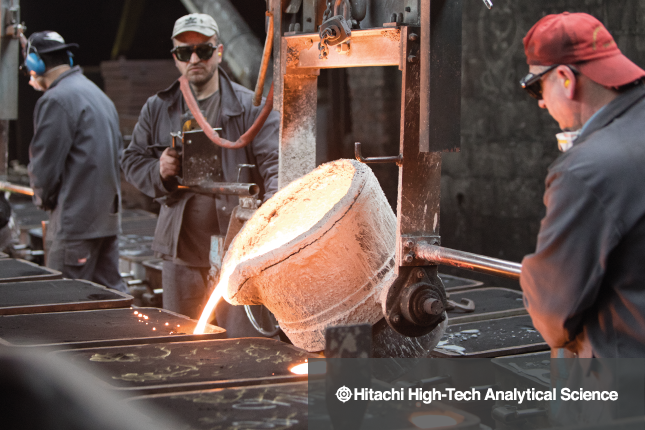# 直读光谱仪（OES）——满足低合金钢规范的最快、最佳选择## 生产可靠性

1. 避免等离子体激发期间氧化

2. 为紫外线范围内的波长创造洁净度（用于碳、氮、硼、磷和其他元素）。氩气对于这些谱线呈透明，而空气会吸收波长200nm以下的谱线。

• 碳当量：CE=(%C)+((%Mn)/6)+(((%Cr)+(%Mo)+(%V))/5)+(((%Cu)+(%Ni))/15)

• 碳当量：CEM=(%C)+((%Si)/25)+(((%Mn)+(%Cu))/20)+(((%Cr)+(%V))/10)+((%Mo)/15)+((%Ni)/40)

• 碳当量：CET =(%C)+(((%Mn)+(%Mo))/10)+(((%Cr)+(%Cu))/20)+((%Ni)/40)

• 碳当量：CEV=(%C)+((%Mn)/6)+(((%Cu)+(%Ni))/15)+(((%Cr)+(%Mo)+(%V))/5)

• 碳当量：PCM=(%C)+((%Si)/30)+(((%Cr)+(%Cu)+(%Cr))/20)+((%Ni)/60)+((%Mo)/15)+((%V)/10)+((%B)*5)

OES为何是降低高合金钢风险的最佳技术

OES仍然是低合金钢分析的最佳之选，原因在于它能够提供100%化学成分，同时检测微量元素和残余元素的检出限极低。另外，它还可以检测轻元素（如碳、氮、磷和硼），最高可精确至ppm水平。当赌注如此之高时，你愿意冒这个险吗?